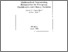Mathematical Programming Formulations for Two-group Classification with Binary Variables

Asparoukhov, O.K. & Stam, A. (1996). Mathematical Programming Formulations for Two-group Classification with Binary Variables. IIASA Working Paper. IIASA, Laxenburg, Austria: WP-96-092Preview
Text
WP-96-092.pdf

Abstract

In this paper, we introduce a nonparametric mathematical programming (MP) approach for solving the binary variable classification problem. In practice, there exists a substantial interest in the binary variable classification problem. For instance, medical diagnoses are often based on the presence or absence of relevant symptoms, and binary variable classification has long been used as a means to predict (diagnose) the nature of the medical condition of patients. Our research is motivated by the fact that none of the existing statistical methods for binary variable classification -- parametric and nonparametric alike -- are fully satisfactory.

The general class of MP classification methods facilitates a geometric interpretation, and MP-based classification rules have intuitive appeal because of their potentially robust properties. These intuitive arguments appear to have merit, and a number of research studies have confirmed that MP methods can indeed yield effective classification rules under certain non-normal data conditions, for instance if the data set is outlier-contaminated or highly skewed. However, the MP-based approach in general lacks a probabilistic foundation, an ad hoc assessment of its classification performance.

Our proposed nonparametric mixed integer programming (MIP) formulation for the binary variable classification problem not only has a geometric interpretation, but also is consistent with the Bayes decision theoretic approach. Therefore, our proposed formulation possesses a strong probabilistic foundation. We also introduce a linear programming (LP) formulation which parallels the concepts underlying the MIP formulation, but does not possess the decision theoretic justification.

An additional advantage of both our LP and MIP formulations is that, due to the fact that the attribute variables are binary, the training sample observations can be partitioned into multinomial cells, allowing for a substantial reduction in the number of binary and deviational variables, so that our formulation can be used to analyze training samples of almost any size.

We illustrate our formulations using an example problem, and use three real data sets to compare its classification performance with a variety of parametric and nonparametric statistical methods. For each of these data sets, our proposed formulation yields the minimum possible number of misclassifications, both using the resubstitution and the leave-one-out method.

Item Type: Monograph (IIASA Working Paper) Institute Scholars (INS) IIASA Import 15 Jan 2016 02:07 27 Aug 2021 17:15 http://pure.iiasa.ac.at/4935View Item